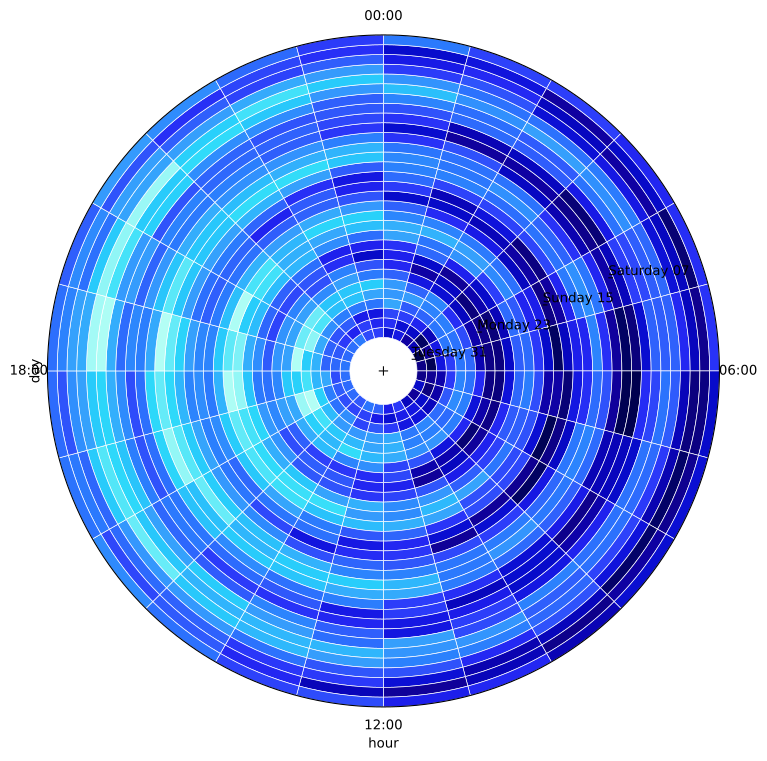Title
Dependencies
Matplotlib
Backends
Matplotlib
Bokeh
```import numpy as np
import pandas as pd
import holoviews as hv
from holoviews import opts

hv.extension('matplotlib')

hv.output(fig='svg')
opts.defaults(opts.HeatMap(fig_size=250))
```A radial `HeatMap` is well suited to discover periodic patterns and trends in time series data and other cyclic variables. A radial HeatMap can be plotted simply by activating the `radial` plot option on the `HeatMap` element.

Here we will create a synthetic dataset of a value varying by the hour of the day and day of the week:

```days = 31
hours = 24
size = days*hours

def generate_hourly_periodic_data(x):
periodic_weekly = np.sin(x*2*np.pi / (24*7))
periodic_daily = np.sin(x*2*np.pi / 24)
noise = np.random.random(size=x.size)
return periodic_weekly + periodic_daily + noise

x = np.linspace(0, size, size)
y = generate_hourly_periodic_data(x)

date_index = pd.date_range(start="2017-10-01", freq="h", periods=size)
kdim_segment = date_index.strftime("%H:%M")
kdim_annular = date_index.strftime("%A %d")

df = pd.DataFrame({"values": y, "hour": kdim_segment, "day": kdim_annular}, index=date_index)
```

As with a regular `HeatMap` the data should consist of two index variables or key dimensions and one or more value dimensions. Here we declare the ‘hour’ and ‘day’ as the key dimensions. For a radial HeatMap to make sense the first key dimension, which will correspond to the radial axis, should be periodic. Here the variable is ‘hour’, starting at midnight at the top:

```hv.HeatMap(df, ["hour", "day"]).opts(radial=True)
```The resulting plot is quite bare so we may want to customize it, there are a number of things we can do to make the plot clearer:

1. Increase the inner padding with the `radius_inner` option.

2. Increase the number of ticks along the radial axis using `xticks`

3. Add radial separator marks with the `xmarks` option.

4. Change the colormap using the `cmap` style option.

```hv.HeatMap(df, ["hour", "day"]).opts(